# 3.6. Scatter Plot

The window Scatter plot displays pixel values for two raster bands as points in the 2D space. Scatter plots are useful for assessing ROI separability between two bands.

The functions are described in detail in the following paragraphs.

## 3.6.1. Scatter list

The Scatter list is a table that includes the spectral signatures added from the SCP dock.

S

MC ID

MC Name

C ID

C Name

Color

checkbox field; if checked, the spectral signature is displayed in the plot

signature Macroclass ID

signature Macroclass Name

signature Class ID

signature Class Name

signature color

The following tools are available.

Tool symbol and name

Description

X band of the plot

Y band of the plot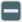remove highlighted signatures from this list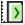add highlighted spectral signatures to ROI & Signature list

use custom precision for calculation (precision should be selected according significant digits): * 4 = $$10^{-4}$$ * 3 = $$10^{-3}$$ * 2 = $$10^{-2}$$ * 1 = $$10^{-1}$$ * 0 = 1 * -1 = 10 * -2 = $$10^{2}$$ * -3 = $$10^{3}$$

calculate the scatter plot for the ROIs checked in the listremove highlighted signatures from this list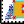add a temporary scatter plot to the list (as MC Name = tempScatter) and start the plot calculation of the last temporary ROI (see Working toolbar)

Warning

Using a precision value that is too high can result in slow calculation or failure.

### 3.6.1.1. Plot

Left click and hold inside the plot to move the view of the plot. Use the mouse wheel to zoom in and out the view of the plot. Right click and hold inside the plot to zoom in a specific area of the plot.

Tool symbol and name

Description

select a colormap that is applied to highlighted scatter plots in the list when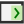is clicked; if no scatter plot is highlighted then the colormap is applied to all the scatter plots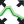automatically fit the plot to data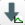save the plot image to file (available formats are .jpg, .png, and .pdf)

x y

display x y coordinates of mouse cursor inside the plot